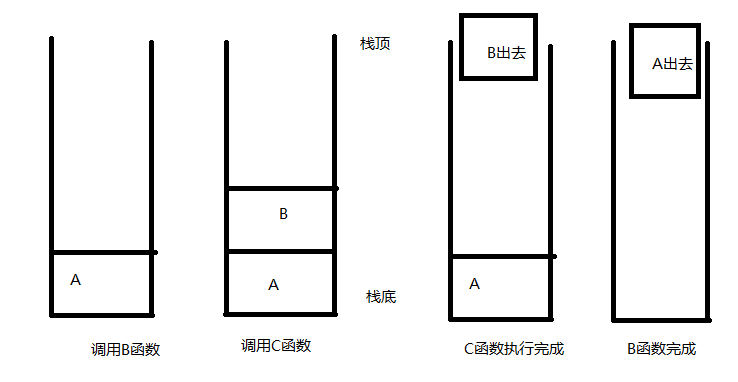# 数据结构学习之栈

public void A()
{
B();
}

public void B()
{
C();
}

public void C()
{
//....
}

A为我们的主函数，当A调用B的时候，进入B函数时，会在A函数中断的位置把函数地址信息压入到系统栈里。/// <summary>
/// 栈
/// </summary>
/// <typeparam name="T"></typeparam>
public interface IStack<T>
{
void Push(T value);
T Pop();
T Peek();
bool IsEmpty();
void Clear();
}

public class Stack<T> : IStack<T>
{
private Array<T> Data;
public Stack(int capacity=20)
{
this.Data = new Array<T>(capacity);
}

public void Clear()
{
this.Data.SetEmpty();
}

public bool IsEmpty()
{
return this.Data.GetSize() == 0;
}

public T Peek()
{
return this.Data.Search(this.Data.GetSize());
}

public T Pop()
{
return this.Data.Delete(this.Data.GetSize());
}

public void Push(T value)
{
}
}

public class Solution {
public bool IsValid(string str) {
var stack = new Stack();
for (int i = 0; i < str.Length; i++)
{
if (str[i] == '[' || str[i] == '{' || str[i] == '(')
stack.Push(str[i]);
else if(str[i] == ']' || str[i] == '}' || str[i] == ')')
{
if (stack.Count == 0)
return false;
var top = (char)stack.Pop();
if (str[i] == ')' && top != '(')
return false;
if (str[i] == ']' && top != '[')
return false;
if (str[i] == '}' && top != '{')
return false;
}
}
return stack.Count==0;
}
}
使用的Stack类为我们的C#本身为我们提供的栈结构。本题目是 [，(,,{ 三种括号的匹配。我们判断。如果是这三种，我们就相应的入栈。当遇到他们所匹配的结尾括号时，],),}时候。便相应的出栈。如果不是他们对应的标签。便是不符合要求的。当一一遍历出栈后。如果栈为空时。便说明便是符合要求的。今天我们的栈就到这里啦，下一次，我们来实现另一种线性结构,队列。

posted @ 2019-06-18 22:48  MUYIgUAN  阅读(196)  评论(0编辑  收藏